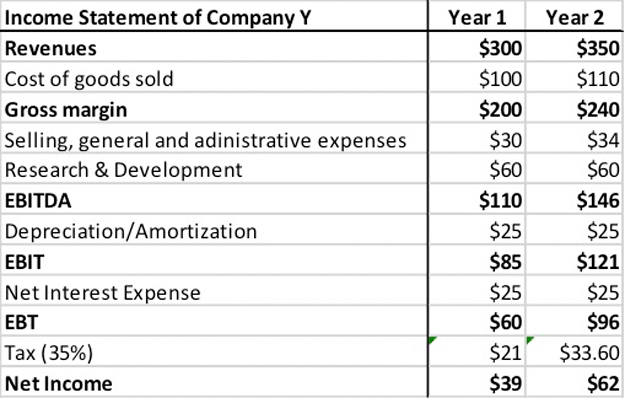# What is Net Operating Profit After Taxes (NOPAT)?

Definition: Net operating profit after taxes (NOPAT) represents a company’s earnings that could be distributed to its shareholders if it had no debt. In other words, it’s the amount of cash earnings left over after all operating expenses and taxes have been paid for the year.

## What Does Net Operating Profit After Tax Mean?

NOPAT is used for the calculation of a firm’s free cash flow (FCF) and economic value added (EVA).

Firms engage in debt and equity financing to fund their operating and non-operating assets. The difference between the revenues and expenses is the firm’s operating income or EBIT (earnings before interest and tax). NOPAT assumes that the firm cannot claim the tax benefits of its debt and adjusts EBIT for taxes. There are two ways to calculate this formula.

The first one is by using the EBIT is calculated like this:

NOPAT = EBIT x ( 1 – Tax Rate )

The second equation uses net income like this:

NOPAT = Net Income + Net Interest Expense x ( 1 – Tax Rate ).

Let’s look at an example.

## Example

Jacob is a finance associate at a boutique securities firm. His manager asked Jacob to calculate the net operating profit after tax of a security using both the EBIT and the net income as portrayed on the company’s income statement.

The company’s income statement for year 1 and for year 2 is as follows:Based on the above table, Year 1 is calculated like this:

EBIT x ( 1 – tax rate ) = \$85 x ( 1 – 35% ) = \$55

Year 2 is calculated like this:

EBIT x ( 1 – tax rate ) = \$121 x ( 1 – 35% ) = \$79.

Then, Jacob compares his findings by calculating it using net income. In this case, Year 1 is calculated like this:

Net Income + Net Interest Expense x (1 – Tax Rate) = \$39 + \$25 x ( 1 – 35% ) = \$55

Year 2 is calculated like this:

Net Income + Net Interest Expense x ( 1 – Tax Rate ) = \$62 + 25 x ( 1 – 35% ) = 79.